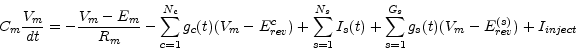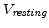CbNeuronSt
Subsections

## CbNeuronSt

### The Model

The membrane voltage Vm is governed bywith the following meanings of symbols
• Em reversal potential of the leak current (Volts)
• Rm membrane resistance (Ohm)
• Nc total number of channels (active + synaptic)
• gc(t) current conductance of channel c (Siemens)
• Erevc reversal potential of channel c (Volts)
• Ns total number of current supplying synapses
• Is(t) current supplied by synapse s (Ampere)
• Gs total number of coductance based synapses
• gs(t) coductance supplied by synapse s (Siemens)
• Erev(s) reversal potential of synapse s (Volts)
• Iinject injected current (Ampere)

At time t=0 Vm ist set to Vinit .

The value of Em is calculated to compensate for ionic currents such that Vm actually has a resting value of.

### Spiking and reseting the membrane voltage

If the membrane voltage Vm exceeds the threshold Vtresh the CbNeuronSt sends a spike to all its outgoing synapses and the membrane voltage follows a predefined spike templage during the absolute refractory period of length Trefract if doReset = 1.

If the flag doReset=0 the spike template is not applied and the above equation is also applied during the absolute refractory period but the event of threshold crossing is transmitted as a spike to outgoing synapses. This is usfull if one includes channels which produce a real action potential (see HH_K_Channel and HH_Na_Channel) but one still just wants to communicate the spikes as events in time.

### Implementation

The exponential Euler method is used for numerical integration.

STempHeight (Volt) :
Height
Vthresh (V) :
If Vm exceeds Vthresh a spike is emmited.
Vreset (V) :
The voltage to reset Vm to after a spike.
doReset (flag) :
Flag which determines wheter Vm should be reseted after a spike
Trefract (sec) :
Length of the absolute refractory period.
nummethod (flag) :
Numerical method for the solution of the differential equation: Exp. Euler = 0, Crank-Nicolson = 1
type :
Type (e.g. inhibitory or excitatory) of the neuron
Cm (F) :
The membrane capacity Cm
Rm (Ohm) :
The membrane resistance Rm
Vresting (V) :
The resting membrane voltage.
Vinit (V) :
Initial condition forVm at time t=0.
VmScale (V) :
Defines the difference between Vresting and the Vthresh for the calculation of the iongate tables and the ionbuffer Erev.
Inoise (W2) :
Variance of the noise to be added each integration time constant.
Iinject (A) :
Constant current to be injected into the CB neuron.

Em (V) :
The reversal potential of the leakage channel
Vm (V) :
The membrane voltage
Isyn :
synaptic input current
Gsyn :
synaptic input conductance
nIncoming :
Number of incoming synapses
nOutgoing :
Number of outgoing synapses
nBuffers :
Number of ion buffers
nChannels :
Number of channels